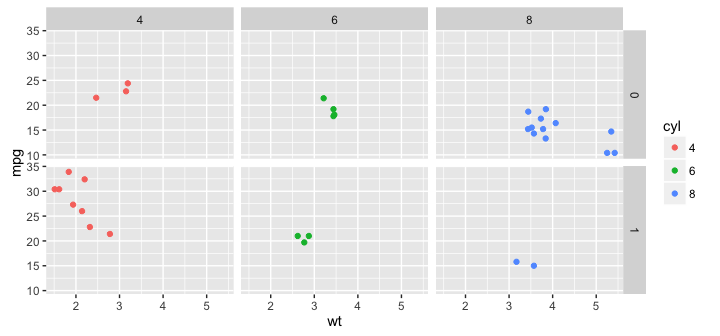## What is ggplot

Useful tutorial from Harvard here

"ggplot2 is a plotting system for R, based on the grammar of graphics, which tries to take the good parts of base and lattice graphics and none of the bad parts. It takes care of many of the fiddly details that make plotting a hassle (like drawing legends) as well as providing a powerful model of graphics that makes it easy to produce complex multi-layered graphics." - http://ggplot2.org

• install.packages("ggplot2")
• Or use the menu option
• We'll also use dplyr (a package to help manipulate data)
• install.packages(dplyr)
library(dplyr); library(ggplot2)

## Illustrating ggplot

• Again, we'll use mtcars
# make cylinders an ordinal factor:
mtcars <- mutate(mtcars,cyl=factor(cyl,ordered=TRUE,levels=c(4,6,8)))
head(mtcars,n=6)
##    mpg cyl disp  hp drat    wt  qsec vs am gear carb
## 1 21.0   6  160 110 3.90 2.620 16.46  0  1    4    4
## 2 21.0   6  160 110 3.90 2.875 17.02  0  1    4    4
## 3 22.8   4  108  93 3.85 2.320 18.61  1  1    4    1
## 4 21.4   6  258 110 3.08 3.215 19.44  1  0    3    1
## 5 18.7   8  360 175 3.15 3.440 17.02  0  0    3    2
## 6 18.1   6  225 105 2.76 3.460 20.22  1  0    3    1

## Histogram

ggplot(mtcars,aes(x=mpg)) + geom_histogram(binwidth=5)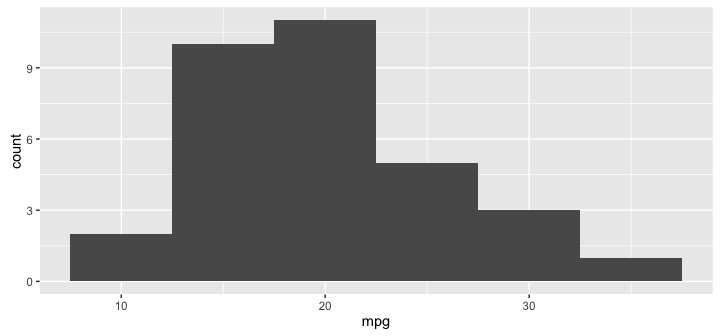• aes = aesthetic here meaning a mapping between a variable and a characteristic of the plot
• x relates to the x-axis (ie mpg links to x)

## What it all means

• ggplot(mtcars,aes(x=mpg)) + geom_histogram(binwidth=5)
• a ggplot object is a list of characteristics of a plot
• + adds to or modifies the characteristics
• ggplot(mtcars,aes(x=mpg)) just creates an empty ggplot object based on mtcars
• geom_histogram(...) adds a histogram to the list of characteristics
• You can store the objects:
my_plot <- ggplot(mtcars,aes(x=mpg)) + geom_histogram(binwidth=5)

• labs adds labels to a plot
my_plot + xlab('Miles per Gallon')+ylab('Number of Cars')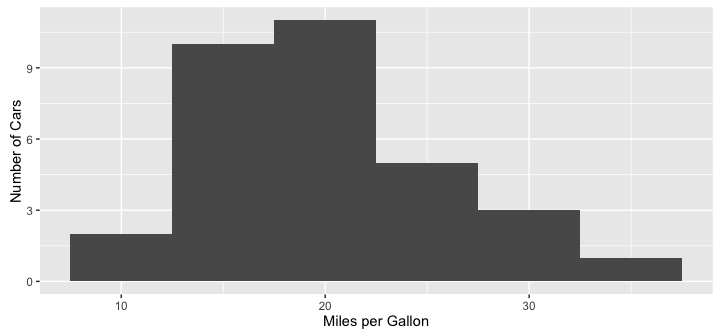• theme_... modifies the 'theme' of a plot
my_plot + xlab('Miles per Gallon')+ylab('Number of Cars')  + theme_dark()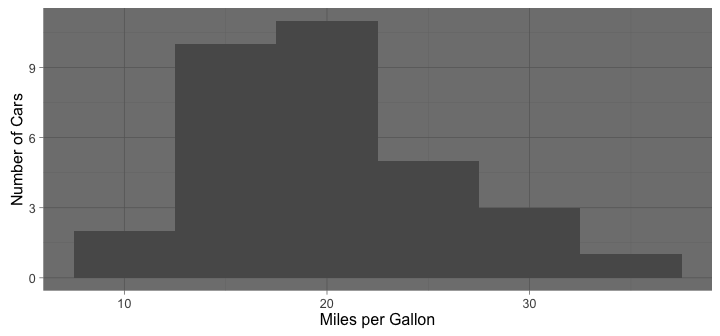• theme_... modifies the 'theme' of a plot
my_plot + xlab('Miles per Gallon')+ylab('Number of Cars') + theme_light()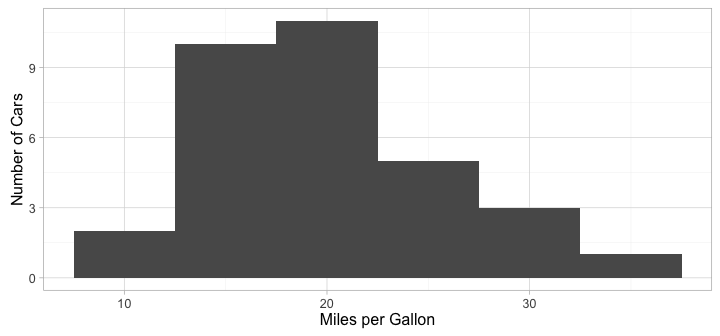## Another neat package is ggthemes

library(ggthemes) # install first if necessary
my_plot + xlab('Miles per Gallon')+ylab('Number of Cars') + theme_economist_white()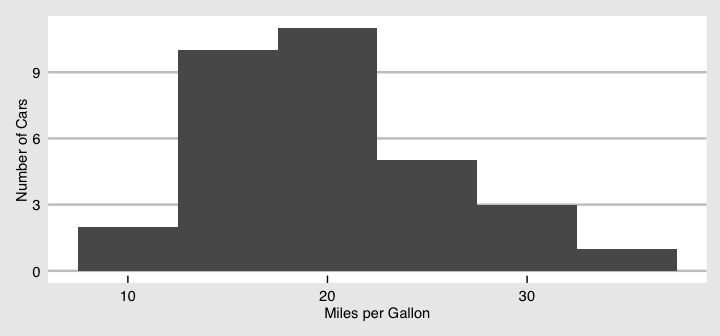## Boxplot

my_boxplot <- ggplot(mtcars,aes(x=cyl,y=mpg)) + geom_boxplot() + xlab('Cylinders') + ylab('Miles per Gallon')
my_boxplot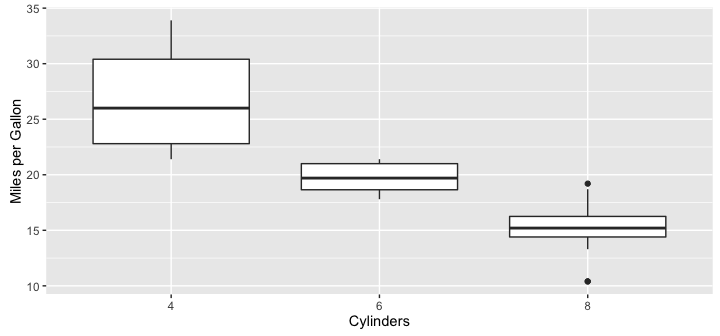• a geom is a geometrical representation of the information - such as a histogram or boxplot

## coord characteristics

• These modify the coordinate system
my_boxplot + coord_flip()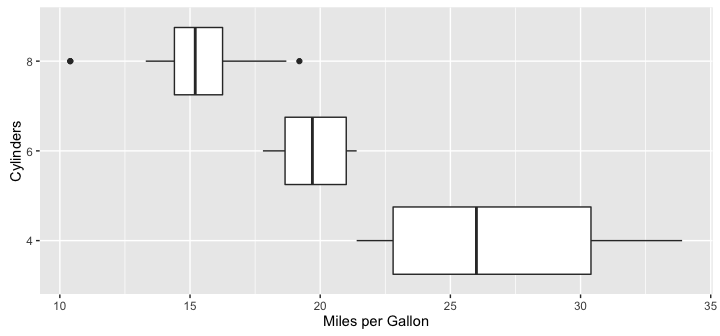## Relationships: Scatterplot

my_scatplot <- ggplot(mtcars,aes(x=wt,y=mpg)) + geom_point()
my_scatplot + xlab('Weight (x 1000lbs)') + ylab('Miles per Gallon')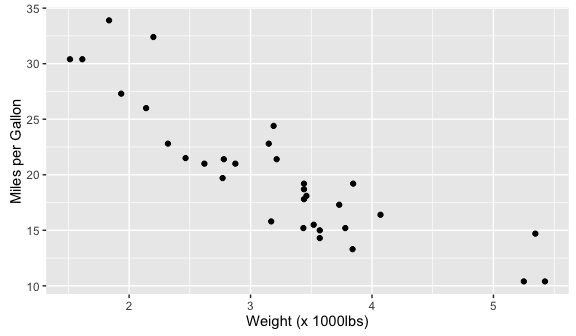## Relationships: Scatterplot

my_scatplot <- ggplot(mtcars,aes(x=wt,y=mpg)) + geom_point()
my_scatplot + xlab('Weight (x 1000lbs)') + ylab('Miles per Gallon') + geom_smooth()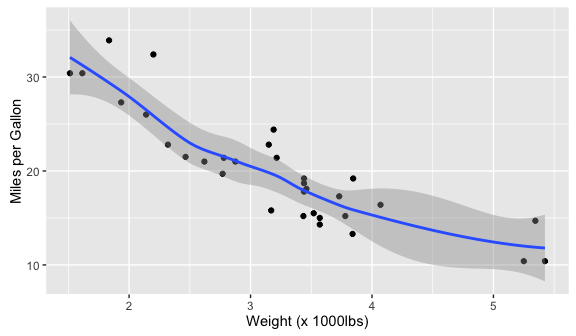## Other Aesthetics

my_scatplot <- ggplot(mtcars,aes(x=wt,y=mpg,col=cyl)) + geom_point()
my_scatplot + labs(x='Weight (x1000lbs)',y='Miles per Gallon',colour='Number of\n Cylinders')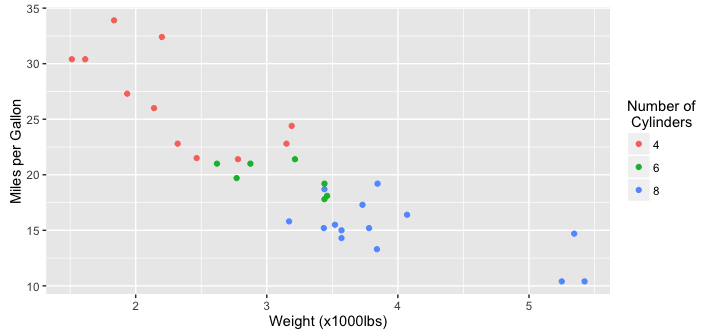## Facets (small multiples)

my_scatplot <- ggplot(mtcars,aes(x=wt,y=mpg,col=cyl)) + geom_point()
my_scatplot + facet_grid(~cyl)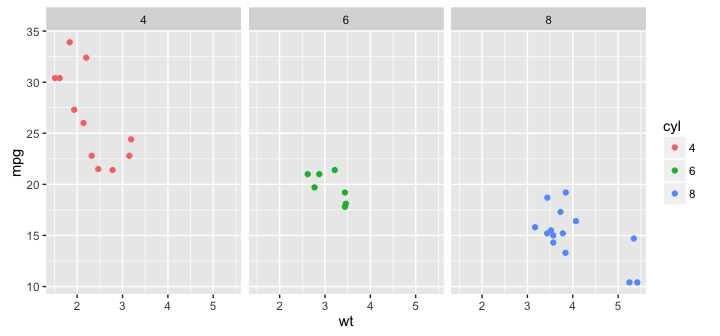## Facets (small multiples)

my_scatplot <- ggplot(mtcars,aes(x=wt,y=mpg,col=cyl)) + geom_point()
my_scatplot + facet_grid(am~cyl)